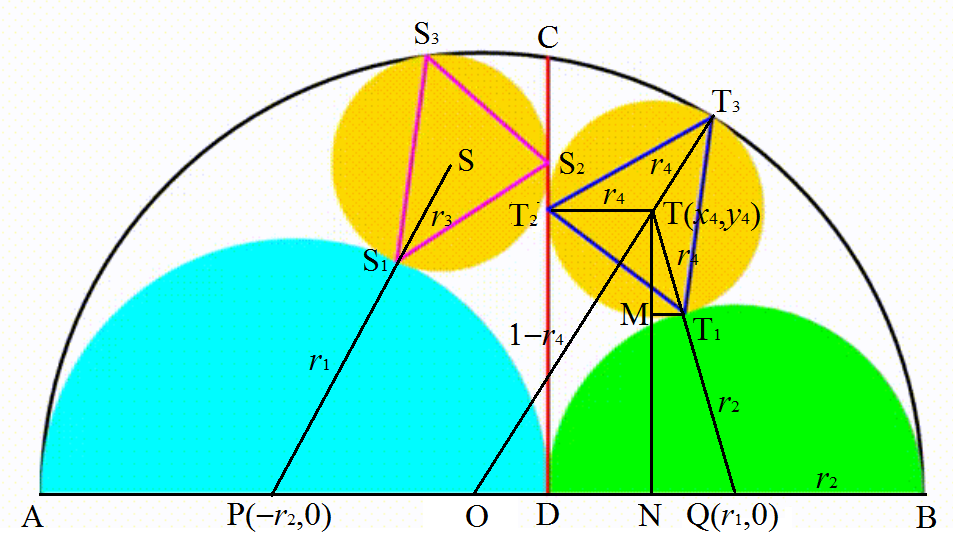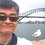# Dynamic Geometry: P32 Series

This note is a compilation of calculations needed in the solutions to the problems titled Dynamic Geometry: P32, 77, 89, 94, and 98 so far by @Valentin Duringer.Let the center of the large semicircle be $O(0,0)$, the origin of the $xy$-plane, its radius $1$, if it is not given, and its diameter $AB$. Let the center and radius of the cyan semicircle be $P$ and $r_1$ and those of the green semicircle be $Q$ and $r_2$. Then $2r_1+2r_2 = AB = 2 \implies r_1 + r_2 = 1 \implies r_2 = 1 - r_1$, $P=(-1+r_1, 0) = (-r_2, 0)$, and $Q = (1-r_2, 0) = (r_1, 0)$.

Let the center and radius of the left yellow circle be $S(x_3, y_3)$ and $r_3$, those of the right yellow circle be $T(x_4, y_4)$ and $r_4$, $CD$ be the vertical dividing red segment, $T_2T$ be perpendicular to $CD$, and $TN$ be perpendicular to $AB$. Then by Pythagorean theorem,

\begin{aligned} TN^2 & = TQ^2 - NQ^2 = TQ^2 - (DQ-T_2T)^2 \\ \implies y_4^2 & = (r_2+r_4)^2 - (r_2-r_4)^2 = 4r_2r_4 \end{aligned}

Also

\begin{aligned} TO^2 - ON^2 & = TN^2 \\ TO^2 - (OQ-NQ)^2 & = TN^2 \\ TO^2 - (OQ-(DQ-T_2T))^2 & = TN^2 \\ (1-r_4)^2 - (r_1 - r_2 + r_4)^2 & = y_4^2 \\ (r_1 + r_2 - r_4)^2 - (r_1 - r_2 + r_4)^2 & = 4r_2r_4 \\ 2r_1(2r_2-2r_4) & = 4r_2r_4 \\ r_1r_2 - r_1r_4 & = r_2r_4 \\ r_1r_2 & = (\blue{r_1+r_2}) r_4 & \small \blue{\text{Note that }r_1+r_2 = 1} \\ \implies r_4 & = r_1r_2 \end{aligned}

Since if we flip the yellow circles and the semicircles below them horizontally about $CD$, $r_1$ becomes $r_2$ and $r_3$ becomes $r_4$. We get the equation for $r_3$ by swapping $r_1$ and $r_2$. Then $r_3 = r_2r_1 = r_4$. Similarly, $y_4 = 2\sqrt{r_2r_4} = 2r_2\sqrt{r_1} \implies y_3 = 2r_1\sqrt{r_2}$. And $x_4 = ON = OQ-(DQ-T_2T) = r_1-r_2+r_4 = r_1-r_2 + r_1r_2 = r_1 - r_2^2 \implies x_3 = r_2 - r_1^2$.

In summary:

$\begin{cases} O(0,0) & 1 \\ P(-r_2,0) & r_1 \\ Q(r_1, 0) & r_2 = 1 - r_1 \\ S(r_2-r_1^2, 2r_1\sqrt{r_2}) & r_1r_2 \\ T(r_1-r_2^2, 2r_2\sqrt{r_1}) & r_1r_2 \end{cases}$

## Vertices of Triangles

Let $S_1(x_{31}. y_{31})$, $S_2(x_{32}. y_{32})$, and $S_3(x_{33}. y_{33})$ be the points where the left yellow circle tangent to the semicircle below it, the segment $CD$, and the unit semicircle respectively. Let the corresponding points of the right yellow circle be $T_1(x_{41}. y_{41})$, $T_2(x_{42}. y_{42})$, and $T_3(x_{43}. y_{43})$ respectively.

Now let $MT_1$ be perpendicular to $TN$. Then

$\begin{cases} x_{41} =x_4+MT_1 = x_4+ \dfrac {r_4(r_1-x_4)}{r_2+r_4} = \dfrac {2r_1-r_2}{1+r_1} \\ y_{41} = \dfrac {r_2y_4}{r_2+r_4} = \dfrac {2r_2\sqrt{r_1}}{1+r_1} \end{cases} \\ \begin{cases} x_{42} = OD = r_1 - r_2 \\ y_{42} = y_4 = 2r_2\sqrt{r_1} \end{cases} \\ \begin{cases} x_{43} = \dfrac {x_4}{1-r_4} = \dfrac {r_1-r_2^2}{1-r_1r_2} \\ y_{43} = \dfrac {y_4}{1-r_4} = \dfrac {2r_2\sqrt{r_1}}{1-r_1r_2} \end{cases}$

Again we can find the coordinates of $S_1$, $S_2$, and $S_3$ by swapping $r_1$ and $r_2$. So in summary:

$\begin{cases} S_1 \left(\dfrac {2r_2-r_1}{1+r_2}, \dfrac {2r_1\sqrt{r_2}}{1+r_2}\right), & T_1 \left(\dfrac {2r_1-r_2}{1+r_1}, \dfrac {2r_2\sqrt{r_1}}{1+r_1} \right) \\ S_2 \left(r_2 - r_1, 2r_1\sqrt{r_2} \right), & T_2 \left(r_1 - r_2, 2r_2\sqrt{r_1} \right) \\ S_3 \left(\dfrac {r_2-r_1^2}{1-r_1r_2}, \dfrac {2r_1\sqrt{r_2}}{1-r_1r_2} \right), & T_3 \left(\dfrac {r_1-r_2^2}{1-r_1r_2}, \dfrac {2r_2\sqrt{r_1}}{1-r_1r_2} \right) \end{cases}$Note by Chew-Seong Cheong
3 weeks ago

This discussion board is a place to discuss our Daily Challenges and the math and science related to those challenges. Explanations are more than just a solution — they should explain the steps and thinking strategies that you used to obtain the solution. Comments should further the discussion of math and science.

When posting on Brilliant:

• Use the emojis to react to an explanation, whether you're congratulating a job well done , or just really confused .
• Ask specific questions about the challenge or the steps in somebody's explanation. Well-posed questions can add a lot to the discussion, but posting "I don't understand!" doesn't help anyone.
• Try to contribute something new to the discussion, whether it is an extension, generalization or other idea related to the challenge.

MarkdownAppears as
*italics* or _italics_ italics
**bold** or __bold__ bold
- bulleted- list
• bulleted
• list
1. numbered2. list
1. numbered
2. list
Note: you must add a full line of space before and after lists for them to show up correctly
paragraph 1paragraph 2

paragraph 1

paragraph 2

[example link](https://brilliant.org)example link
> This is a quote
This is a quote
    # I indented these lines
# 4 spaces, and now they show
# up as a code block.

print "hello world"
# I indented these lines
# 4 spaces, and now they show
# up as a code block.

print "hello world"
MathAppears as
Remember to wrap math in $$ ... $$ or $ ... $ to ensure proper formatting.
2 \times 3 $2 \times 3$
2^{34} $2^{34}$
a_{i-1} $a_{i-1}$
\frac{2}{3} $\frac{2}{3}$
\sqrt{2} $\sqrt{2}$
\sum_{i=1}^3 $\sum_{i=1}^3$
\sin \theta $\sin \theta$
\boxed{123} $\boxed{123}$